### TIME Function Examples – Excel, VBA, & Google Sheets

This tutorial demonstrates how to use the Excel TIME Function in Excel to calculate a time.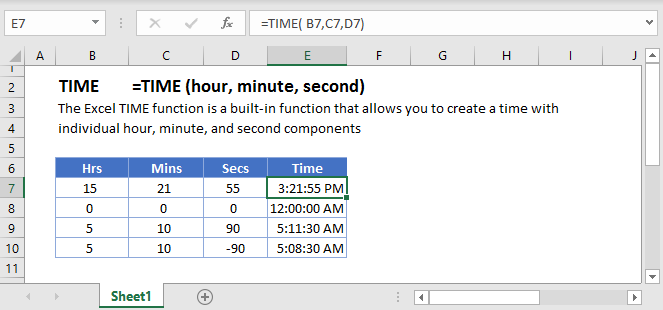## TIME Function Overview

The TIME Function Returns the time from a hour, minute, and second.

To use the TIME Excel Worksheet Function, Select cell and Type: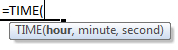(Notice how the formula input appear)

## TIME Function syntax and Inputs:

hour – An hour between 0-23. Example: 13.

minute – A minute between 0-59. Example: 13.

second – An hour between 0-23. Example: 13.

AutoMacro - VBA Code Generator

## TIME Examples

The TIME Function is used to create times by entering a hour, minute, and second: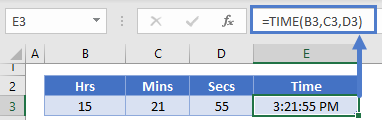### TIME – HOUR, MINUTE, SECOND Functions

Often the TIME Function is used along with HOUR, MINUTE, or SECOND Functions to calculate a time based on another time.

This example will return a time 8 hours after another time: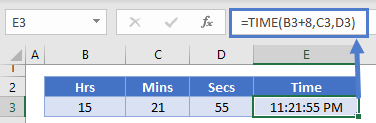### Time – Fraction of Day

TIME values are stored as a decimal value in Excel: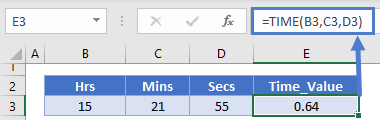## TIME in Google Sheets

The TIME Function works exactly the same in Google Sheets as in Excel: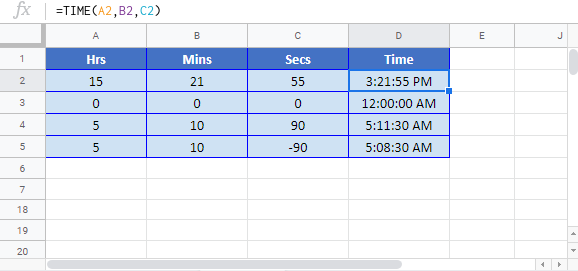## Additional Notes

Simply enter numbers corresponding to the Hour, Minute, and Second of the time.

Minutes and seconds can be greater than 59 or less than 0.

=time(8,0,0) returns 8:00:00 AM.
From this starting point you can add or subtract minutes or seconds.

=time(8,-1,-1) returns 7:58:59 AM.

=time(8,61,61) returns 9:02:01 AM.

The result of the TIME Function may appear as a decimal number. Change the cell Number Formatting to TIME to display the time:## TIME Examples in VBA

You can also use the TIME function in VBA. Type:
`application.worksheetfunction.time(hour,minute,second)`
For the function arguments (hour, etc.), you can either enter them directly into the function, or define variables to use instead.

Return to the List of all Functions in Excel

## Excel Practice Worksheet

Practice Excel functions and formulas with our 100% free practice worksheets!

• Automatically Graded Exercises
• Learn Excel, Inside Excel!